085-1263404

### My Journal

All things Mathematical
20 Sep 2018
##### Question 1

A body of mass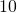units moves in a straight line so that its distance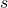from a fixed point in the line of motion at timeis given by the equation :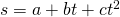.

Derive formulae for its velocity, acceleration and momentum at any time.

Given that when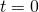the velocity, acceleration and momentum at any time.

Given that whenthe velocity in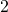units and that the distances travelled when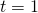, and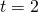are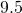and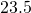respectively, determine the values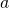,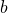and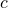in the above equation.

##### Question 2

A mass weighington drops without rebound from a height of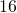ft. on to the top of a pile weighing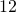cwt., and the pile is driven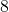inches into the ground by the blow.

Calculate in ft.lbs. the energy lost in the impact, and the average resistance offered by the ground to the motion of the pile.

##### Question 3

Calculate the effective h.p. delivered by the engine of aton motor vehicle in each of the following cases :-

(a) The vehicle is ascending an incline ofin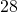with a uniform speed of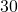miles per hour;

(b) the vehicle accelerates uniformly from a speed of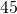miles per hour to a speed of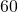miles per hour while travelling a distance of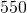yards along a level road.

The frictional resistances to motion may be taken as the same in both cases and equal to a force oflbs. wt.

##### Question 4

State the laws of statical friction.

A uniform ladder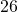feet long and weighing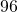lbs. is on the point of slipping when placed with its upper end against a smooth vertical wall and its lower end on a rough horizontal surface at a distance offeet from the wall.

Calculate the value of the frictional force between the foot of the ladder and the ground and also the value of the coefficient of friction.

##### Question 5

A point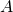describes a circular path of radius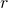with a uniform velocity.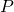is the foot of the perpendicular fromto a fixed diameter of the circle. Show that the velocity and acceleration ofat any instant are equal to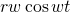and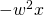respectively, where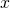is the displacement offron [sic] the centre of the circle,is the time for this displacement and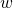is the angular velocity of.

(b) Show that for small oscillations the bob of a simple pendulum may be regarded as having a similar motion of that ofand that the time of oscillation is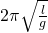whereis the length of the pendulum.

##### Question 6

Through what vertical height will the bob of a conical pendulum drop if its speed falls fromtorevolutions per minute?

##### Question 7

A train is travelling at the rate ofmiles per hour round a circular track of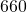yards radius. The horizontal distance between the rails is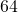inches. How much must the outer rail be raised [above the inner one so that there may be no side thrust on the rails ?

##### Question 8

The force acting on a body moving in a straight line is given at any instant by the formula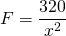where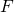is in lbs.-st. andis the distance in feet of the body at that instant from a fixed point in the line. If the motion is resisted by a constant force of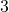lbs.-wt., find the kinetic energy stored in the body in moving from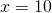to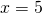.

##### Question 9

A particle of mass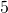lbs. is placed on a smooth plane inclined at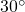to the horizon and is connected by a light string passing over the top of the plane to a particle weighinglbs. which hangs vertically.

Find (a) the common acceleration;

(b) the velocity afterseconds of the centre of inertia of the particles.

##### Question 10

A body is projected up a smooth incline ofwith an initial velocity offeet per second. If the length of the incline is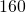feet find the greatest height attained by the body and the range on a horizontal plane passing through the foot of the incline.

##### Citation:

State Examinations Commission (2018). State Examination Commission. Accessed at: https://www.examinations.ie/?l=en&mc=au&sc=ru

Malone, D and Murray, H. (2016). Archive of Maths State Exams Papers. Accessed at: http://archive.maths.nuim.ie/staff/dmalone/StateExamPapers/

##### Licence:

“Contains Irish Public Sector Information licensed under a Creative Commons Attribution 4.0 International (CC BY 4.0) licence”.

The EU Directive 2003/98/EC on the re-use of public sector information, its amendment EU Directive 2013/37/EC, its transposed Irish Statutory Instruments S.I. No. 279/2005, S.I No. 103/2008, and S.I. No. 525/2015, and related Circulars issued by the Department of Finance (Circular 32/05), and Department of Public Expenditure and Reform (Circular 16/15 and Circular 12/16).

Note. Circular 12/2016: Licence for Re-Use of Public Sector Information adopts CC-BY as the standard PSI licence, and notes that the open standard licence identified in this Circular supersedes PSI General Licence No: 2005/08/01.